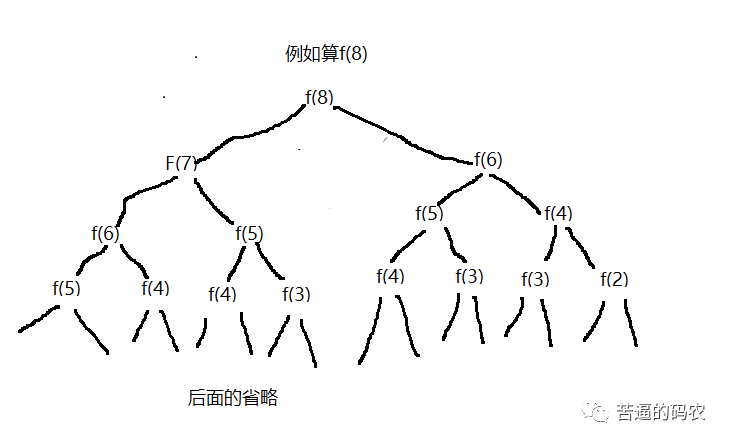# 【带你装逼带你飞】吐血总结了这五大常用算法技巧，让你在同事/面试官面前惊艳全场！

### 1、多思考能否使用位运算

n / 2 等价于 n >> 1
n / 4 等价于 n >> 2
n / 8 等价于 n >> 3。

if( n % 2 == 1){
dosomething();
}


if( (n & 2) == 1){
dosomething();
}


##### 1、利用 n & (n - 1)消去 n 最后的一位 1

n = n & (n - 1)

n = 1001
n - 1 = 1000
n = n & (n - 1) = (1001) & (1000) = 1000

###### （1）、判断一个正整数 n 是否为 2 的幂次方

2^0 = 0…..0001

2^1 = 0…..0010

2^2 = 0….0100

boolean judege(int n){
return (n & (n - 1)) == 0;//
}


###### （2）、判断 正整数 n 的二进制表示中有多少个 1

    public int NumberOf12(int n) {
int count = 0;
int k = 1;
while (n != 0) {
count++;
n = (n - 1) & n;
}
return count;


##### 2、异或(^)运算的妙用

###### 案例1：只出现一次是数

123451234 = （11)(22)(33)(44)5= 00005 = 5。

int find(int[] arr){
int tmp = arr;
for(int i = 1;i < arr.length; i++){
tmp = tmp ^ arr[i];
}
return tmp;
}


### 2、考虑是否可以使用数组下标

public void f(int arr[]) {

int[] temp = new int;
for (int i = 0; i < arr.length; i++) {
temp[arr[i]]++;
}
//顺序打印
for (int i = 0; i < 21; i++) {
for (int j = 0; j < temp[i]; j++) {
System.out.println(i);
}
}
}


4byte * 20亿，一共需要80亿个字节

### 3、考虑能否使用双指针

int find(int arr[], int target){
int left = 0;//头指针
int right = arr.length - 1;// 尾指针
while(left < right){
if(left + right == target){
// 找到目标数，进行打印，这里我就不执行打印操作两
}else if(left + right < target){
left ++;
}else{
right --;
}
}
}


### 4、从递归到备忘录到递推或者动态规划

##### （1）.对于可以递归的问题务必考虑是否有重复计算的

f(n) = f(n-1) + f(n - 2)。

    public int f(int n) {
if (n <= 2) {
return n;
} else {
return f(n - 1) + f(n - 2);
}
}//数组的大小根据具体情况来，由于int数组元素的的默认值是0
int[] arr = new int;
public int f(int n) {
if (n <= 2) {
return n;
} else {
if (arr[n] != 0) {
return arr[n];//已经计算过，直接返回
} else {
arr[n] = f(n-1) + f(n-2);
return arr[n];
}
}
}



##### (2).考虑自底向上

f(1) = 1;
f(2) = 2;

public int f(int n) {
if(n <= 2)
return n;

int f1 = 1;
int f2 = 2;
int sum = 0;

for (int i = 3; i <= n; i++) {
sum = f1 + f2;
f1 = f2;
f2 = sum;
}
return sum;
}



leetcode 的 62 号题：https://leetcode-cn.com/problems/unique-paths/

leetcode 的第64题：https://leetcode-cn.com/problems/minimum-path-sum/

(1). 是否有状态重复计算的，可不可以使用备忘录法来优化。

(2). 是否可以采取递推的方法来自底向上做，减少一味递归的开销。

### 兄dei，如果觉得我写的不错，不妨帮个忙

1、关注我的原创微信公众号「帅地玩编程」，每天准时推送干货技术文章，专注于写算法 + 计算机基础知识（计算机网络+ 操作系统+数据库+Linux），听说关注了的不优秀也会变得优秀哦。

2、给俺点个赞呗，可以让更多的人看到这篇文章，顺便激励下我，嘻嘻。

### 作者简洁

posted @ 2020-02-27 12:17  帅地  阅读(1856)  评论(5编辑  收藏